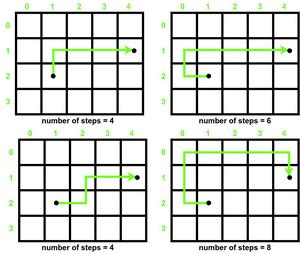# Check if it is possible to reach destination in even number of steps in an Infinite Matrix

• Difficulty Level : Medium
• Last Updated : 11 Aug, 2022

Given a source and destination in a matrix[][] of infinite rows and columns, the task is to find whether it is possible to reach the destination from the source in an even number of steps. Also, you can only move up, down, left, and right.

Examples:

Input: Source = {2, 1}, Destination = {1, 4}
Output: Yes

Input: Source = {2, 2}, Destination = {1, 4}
Output: No

Observation:

The observation is that if the steps required to reach the destination in the shortest path is even, then steps required in every other route to reach it will always be even. Also, there can be an infinite number of ways to reach the target point. Some paths to reach (4, 1) from (1, 2) in a 4 x 5 matrix are given below :Minimum number of steps required =4

So our problem is reduced in finding the minimum number of steps required to reach the destination from source in a matrix, which can be calculated easily by simply taking the sum of the absolute values of the difference between the X coordinates and Y coordinates.Below is the implementation of the above approach:

## C++

 `// C++ Program for the above approach``#include ``using` `namespace` `std;` `// Function to check destination can be``// reached from source in even number of``// steps``void` `IsEvenPath(``int` `Source[], ``int` `Destination[])``{``    ``// Coordinates differences``    ``int` `x_dif = ``abs``(Source - Destination);``    ``int` `y_dif = ``abs``(Source - Destination);` `    ``// minimum number of steps required``    ``int` `minsteps = x_dif + y_dif;` `    ``// Minsteps is even``    ``if` `(minsteps % 2 == 0)``        ``cout << ``"Yes"``;` `    ``// Minsteps is odd``    ``else``        ``cout << ``"No"``;``}` `// Driver Code``int` `main()``{``    ``// Given Input``    ``int` `Source[] = { 2, 1 };``    ``int` `Destination[] = { 1, 4 };` `    ``// Function Call``    ``IsEvenPath(Source, Destination);` `    ``return` `0;``}`

## Java

 `// Java program for the above approach``import` `java.lang.*;``import` `java.util.*;` `class` `GFG{` `// Function to check destination can be``// reached from source in even number of``// steps``static` `void` `IsEvenPath(``int` `Source[], ``int` `Destination[])``{``    ` `    ``// Coordinates differences``    ``int` `x_dif = Math.abs(Source[``0``] - Destination[``0``]);``    ``int` `y_dif = Math.abs(Source[``1``] - Destination[``1``]);` `    ``// Minimum number of steps required``    ``int` `minsteps = x_dif + y_dif;` `    ``// Minsteps is even``    ``if` `(minsteps % ``2` `== ``0``)``        ``System.out.println(``"Yes"``);` `    ``// Minsteps is odd``    ``else``        ``System.out.println(``"No"``);``}` `// Driver code``public` `static` `void` `main(String[] args)``{``    ` `    ``// Given Input``    ``int` `Source[] = { ``2``, ``1` `};``    ``int` `Destination[] = { ``1``, ``4` `};` `    ``// Function Call``    ``IsEvenPath(Source, Destination);``}``}` `// This code is contributed by sanjoy_62`

## Python3

 `# Python3 program for the above approach` `# Function to check destination can be``# reached from source in even number of``# steps``def` `IsEvenPath(Source, Destination):` `    ``# Coordinates differences``    ``x_dif ``=` `abs``(Source[``0``] ``-` `Destination[``0``])``    ``y_dif ``=` `abs``(Source[``1``] ``-` `Destination[``1``])` `    ``# Minimum number of steps required``    ``minsteps ``=` `x_dif ``+` `y_dif` `    ``# Minsteps is even``    ``if` `(minsteps ``%` `2` `=``=` `0``):``        ``print``(``"Yes"``)` `    ``# Minsteps is odd``    ``else``:``        ``print``(``"No"``)` `# Driver Code``if` `__name__ ``=``=` `'__main__'``:``    ` `    ``# Given Input``    ``Source ``=` `[ ``2``, ``1` `]``    ``Destination ``=` `[ ``1``, ``4` `]` `    ``# Function Call``    ``IsEvenPath(Source, Destination)` `# This code is contributed by mohit kumar 29`

## C#

 `// C# program for the above approach``using` `System;` `class` `GFG{` `// Function to check destination can be``// reached from source in even number of``// steps``static` `void` `IsEvenPath(``int``[] Source, ``int``[] Destination)``{``    ` `    ``// Coordinates differences``    ``int` `x_dif = Math.Abs(Source - Destination);``    ``int` `y_dif = Math.Abs(Source - Destination);` `    ``// Minimum number of steps required``    ``int` `minsteps = x_dif + y_dif;` `    ``// Minsteps is even``    ``if` `(minsteps % 2 == 0)``        ``Console.WriteLine(``"Yes"``);` `    ``// Minsteps is odd``    ``else``        ``Console.WriteLine(``"No"``);``}` `// Driver code``public` `static` `void` `Main(``string``[] args)``{``    ``// Given Input``    ``int``[] Source = { 2, 1 };``    ``int``[] Destination = { 1, 4 };` `    ``// Function Call``    ``IsEvenPath(Source, Destination);``}``}` `// This code is contributed by code_hunt.`

## Javascript

 ``

Output

`Yes`

Time Complexity: O(1) // since no loop is used the algorithm takes up constant time to perform the operations
Auxiliary Space: O(1) // since no extra array is used so the space taken by the algorithm is constant

My Personal Notes arrow_drop_up